# What is an Oscilloscope?

The measuring instrument that measures electrical magnitudes and expresses the quantities they measure numerically or analogously is called an oscilloscope.

Most digital oscilloscopes have automatic measuring tools that simplify and speed up common measurement procedures. Thus, the reliability and accuracy of the measurement results have become even better. The manual measurement process described in this article will make the operation and control of automatic measurements more understandable.

When the 12 V AC voltage measured with a Voltmeter is Measured with the Oscilloscope, A Value of Approximately 16.97 V is read the reason of the different values ​​of the measuring instruments is Measuring the effective value of Ac, the Oscilloscope's Measuring the maximum value of Ac.

Although digital oscilloscopes are more expensive than other measuring instruments, it is easier to detect malfunctions in a system with  digital oscilloscopes. Because the outputs of television or more complex systems at certain points and floors are fixed and these outputs are specified point by point in the catalogs of the system. In the measurements made with the oscilloscope, there is a fault on the floor that gives a different output from the catalog.

You may also like : https://compocket.com/blogs/news/how-to-use-an-oscilloscope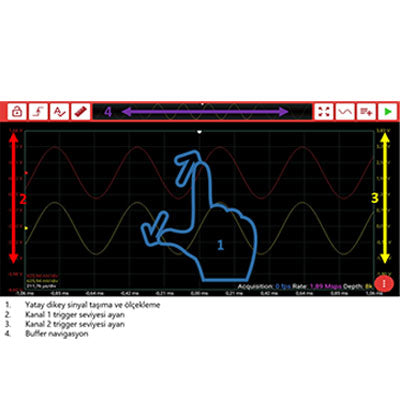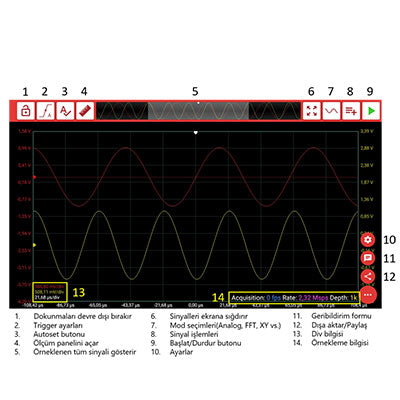## How to start reading an oscilloscope?

You can see the current state of the electrical signal by reading an oscilloscope. Moreover, you can also acquire additional information like the change in the noise of signal over time or the strength of DC (direct current) or AC (alternating current). You can also identify the shape of a wave by reading an oscilloscope.

It may appear very confusing but actually, reading an oscilloscope is much easier than it seems. If you know your way around all the controls and buttons of the device, reading an oscilloscope is just doing some basic mathematics.

First you need to learn what the values on the oscilloscope represent. Each value you see on the oscilloscope informs you about crucial variables like voltage, current strength and so forth.

You can observe almost infinite amounts and varieties of signals with the help of an oscilloscope. Yet different technologies and display options are employed in every oscilloscope, that is why no two oscilloscope reading experiences of yours will be the same. In other words, various types of oscilloscopes offer you some room for creativity and dynamism. However, this does not mean that there is no consistency in different types and designs of oscilloscope. Although they may vary in technology level, style and design, different oscilloscopes show same values for same measurement. That is why there are some essential steps you need to take every time you read an oscilloscope, regardless of the type of the oscilloscope you use.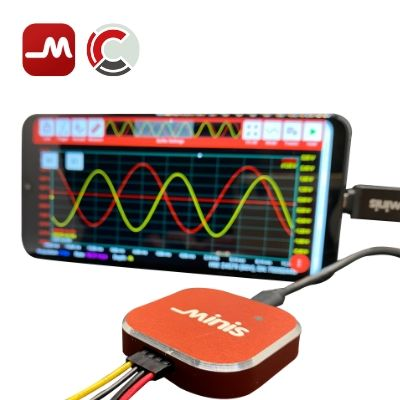Thanks to their improved dependability over the years, digital oscilloscopes offer significant ease of use: They help you bypass the calculation step and just display the results in the screen. However, if you are a student or do not have access to a digital oscilloscope, you need to take additional steps to extract the information you need from the oscilloscope.

On the LCD screen of an oscilloscope, we see x axis and y axis in a wave of rays. Those two helps us determine the potential difference of voltage in time. The length of these waves does not represent the distance, instead they represent time.

The x axis has the y axis on the output screen of the oscilloscope. The x axis shows the time, the y axis shows the voltage. Digital oscilloscopes enable us to determine the relationships between certain variables in electrical circuits.

## How to read an Oscilloscope ?

Oscilloscope is a life saver device for electronics and people who work with electronic currents, circuits. As in all devices, oscilloscopes have some basics to understand it. We know from oscilloscopes, there are many types. However, their types do not change their basic functions. When we ask to how to read an oscilloscope, analysing is depend on a kind of graph screen and this graph has x and y axis. We know from the oscilloscope definition that oscilloscope has time line to measure electrical voltage in the current. X axis is horizontal and it shows time , Y axis is vertical and it shows voltage data. We can see it from the screen. What we are look from them is to detect the problem between these two axises. When you trigger an oscilloscope or when you try to measure what you can measure by using oscilloscope like shape of a signal, measure frequency or amplitude or observing a noise from signal, you see waves on the screen to examine them. There are some common waveforms and their device sources.

• Sine Wave-Electronic Outlets can be source of it.
• Damped Sine Wave
• Square Wave
• Rectangular Wave
• Sawtooth Wave
• Triangle Wave-Automobile can be source of it.
• Step Wav
• Pulse Wave- Computer can be source of it.
• Comlex Wave- Television can be source of it.

By examining these waves, we can reach freaquency, voltage, amplitude, phase knowledge.

## Voltage Measurements

The amount of electrical potential between two points in a circuit is called voltage and its unit is expressed in volts. Alternating current, direct current and high frequency signals can be measured up to a maximum of 400 Volts with the oscilloscope.

The amplitude of the sign on the screen is measured on the Y (vertical) axis. The amplitude is first determined in squares on the screen. Then the actual value of the voltage is determined by multiplying the value indicated by the sign on the Volt / Div input attenuator commutator and the number of frames. At this time, the continuous amplitude adjustment knob, if any, must be turned to the end in the cal position or counterclockwise. If the probe weakens the amplitude; The attenuation coefficient should be multiplied and taken into account.

U = Number of Frames x (Volt / div) * Probe Coefficient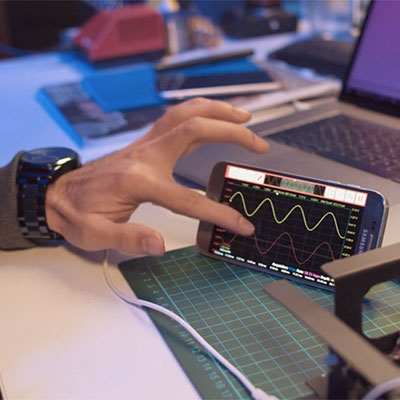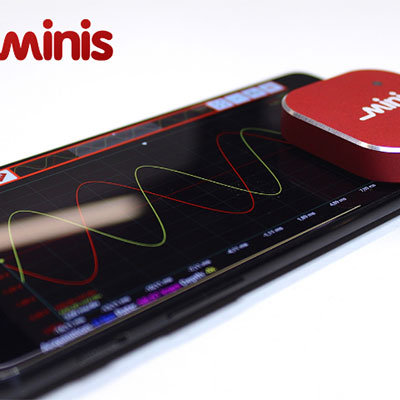## Period or Frequency Measurement

Each digital oscilloscope has a frequency measurement limit. Attention should be paid to this limit when measuring high frequencies. After selecting the digital oscilloscope suitable for the frequency value to be measured, an oscilloscope connection is made to the point to be measured. However, period is now measured in oscilloscopes instead of frequency. Period measurements are made on the X (horizontal) axis. The length of one period of the waveform in the X axis direction is determined by counting the squares. We get the value of the time / div button by multiplying the number of frames. However, if the probe weakens, the attenuation coefficient is multiplied and taken into account.

T = Number of Frames x (Time / div) * Probe Coefficient

### What do oscilloscopes measure?

• AC and DC voltage values
• Waveforms of changing electrical magnitudes
• Current passing through the circuit
• Phase difference
• Frequency
• Characteristics of semiconductor elements such as diodes, transistors
• Charge and discharge curves of the capacitor